# (8b) How distant is the Moon?

Hipparchus, who used an eclipse of the Moon to deduce the precession of the equinoxes (here), used a total eclipse of the Sun--probably in 129 BC--to estimate how far the Moon was. (But he also derived the Moon's distance from a lunar eclipse--see here.)

That eclipse was total at the Hellespont--the Dardanelles, part of the narrow strait that separates the European and Asian parts of Turkey--but only 4/ 5 of the Sun were covered in Alexandria of Egypt, further to the south.

Hipparchus knew that when the Sun was eclipsed, it and the Moon occupied the same spot on the sphere of the heavens. The reason, he assumed, was that the Moon passed between us and the Sun.

He believed that the Sun was much more distant than the Moon, as Aristarchus of Samos had concluded, about a century earlier, from observing the time when the Moon was exactly half full. He also assumed that the peak of the eclipse occured at the same time at both locations (not assured, but luckily not too far off), and he then carried out the following calculation.

## The Eclipse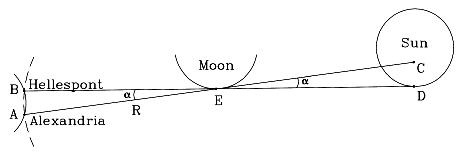In a total eclipse of the Sun, the Moon just barely covers the Sun. The Sun itself is so distant that when viewed from anywhere on Earth, it covers practically the same patch of the sky, with a width of about 0.5°. Hipparchus concentrated on point E at the edge of the Moon (drawing), which during totality, when viewed from the Hellespont (point B) just overlapped point D on the edge of the Sun.

Viewed from Alexandria (point A), at that same moment, the point E only overlapped point C on the Sun, about 1/ 5 solar diameter short of the edge--which was why the eclipse there was not total. One-fifth of the Sun's diameter covers about 0.1° in the sky, so the small angle a (alpha, Greek A) between the two directions measured about 0.1 degrees.

It is unlikely that Hipparchus knew the distance AB, but he probably knew the latitudes of the Hellespont and Alexandria. The local latitude can be shown to be equal to the elevation of the celestial pole above the horizon and today can be readily deduced by observing the height of Polaris, the pole star above the horizon. In the time of Hipparchus the pole of the heavens wasn't near Polaris (because of the precession of the equinoxes), but Hipparchus, who had mapped the positions of about 850 stars, must have known its position quite well.

The latitude of the Hellespont (from a modern atlas) is about 40° 20' (40 degrees and 20 minutes, 60 minutes per degree), while that of Alexandria is about 31° 20', a difference of 9°. We will also assume Alexandria is exactly due south. If furtermore r is the radius of the Earth, then the circumference of the Earth 2p R, where p = 3.1415926... ("pi", Greek lower-case P) is the ratio between the circumference and diameter of any circle. Since the circumference also equals 360°, we get

AB = (2p r/ 360)· 9

where the dot marks multiplication (algebra's equivalent to the x symbol).

## The distance R to the Moon

The points AB are also located on another circle, centered on the Moon. The radius in that case is the distance R to the Moon, and because the arc AB covers 0.1°, we get

AB = (2p R/ 360)·0.1
Strictly speaking, each of the two arcs AB expressed in the above equations is measured along a different circle, with a different radius (and the two circles curve in opposite ways). However, in both cases AB covers only a small part of the circle, so that as an approximation we may regard each of the arcs as equal to the straight-line distance AB. That assumption allows us to regard the two expressions as equal and to write

(2p R / 360)·0.1 = (2p r / 360)·9

Multiplying both sides by 360 and dividing by 2p give

0.1 R   =   9 r
Dividing by (0.1 r)

R/r   =   90

suggesting the Moon's distance is 90 Earth radii, an overestimate of about 50%.

## A more accurate calculation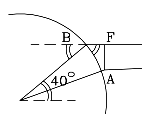One reason an excessive value was obtained is that the Moon was assumed to be overhead at A or B. Actually, it is likely to be at some significant angle to the overhead direction, the "zenith" (see drawing).

Then the section cut by the angle a from the circle of radius R around E is not AB but AF (second drawing), which is smaller. Taking this into account reduces the distance.

We don't know where the Sun was during the 129 BC eclipse, but it must have been on the ecliptic (can you guess how that word originated...?), which places it within 23.5° of the celestial equator, on either side. Assuming it was on the equator (that is, it passed overhead on the Earth's equator) and south of the reported observations (i.e. the eclipse occured near noon) one can make a crude estimate of the correction, using simple trigonometry ( see section M-8).

The Hellespont is around latitude of 40 degrees, and as the drawing shows, that is also the angle between the Moon's direction and the zenith. From the drawing (x marks multiplication)

AF = AB cos 40° = 0.766 AB

Repeating the preceding calculation for AF

AF = (2p R/ 360)·0.1

AF = 0.766 AB = 0.766·(2p r/ 360)·9

and in the end

R/r   =   90·0.766 = 69

According to "A History of Astronomy" by A. Pannekoek, the result obtained by Hipparchus was between 62 and 73 Earth radii. Today we know the average distance is about 60 radii, varying by a few Earth radii either way because of the ellipticity of the Moon's orbit.

In the absence of accurate timing, the method is almost guaranteed to produce an overestimate. The Earth rotates beneath the shadow spot cast by the Moon, which makes that spot sweep over a long strip, hitting many different loactions at different times. The Hellespont was just one of many places where the eclipse was total. Similarly, Alexandria was just one of many locations where 4/5 of the Sun was covered. Randomly selecting point B from the first group and point A from the second may give a much longer baseline AB and a much larger (and incorrect) distance of the Moon. The fact Alexandria is almost exactly south of the Hellespont does not guarantee their peak eclipse timesare the same, just that they are not too different.

### Parallax

An angle like a, representing the shift in the direction of a viewed object when viewed from two slightly different locations, is called its parallax. More about parallaxes--for anyone who wishes to pursue this matter further--is found in the section that follows, linkedat the bottom of this page.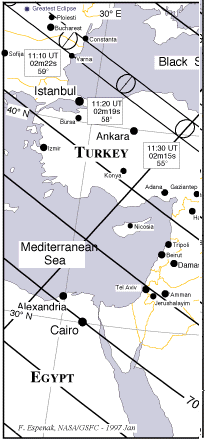### Does history repeat itself?

A total eclipse of the Sun on August 11, 1999, will pass just a few hundred kilometers north of the one which Hipparchus used. Its path of totality extends from the ocean off New England, through England and central Europe, all the way across India. An eclipse map of the relevant area is shown on the right--the double line outlines the region of totality, and the lines parallel to it give locations where 90%, 80% etc of the Sun are covered. As can be seen, the totality path crosses the Black Sea around 11:15 am Universal Time (1:15 pm local time) about 300 km. northeast of the Hellespont, and in Alexandria 71% of the Sun's diameter are covered (rather than 80% as in the Hipparchus eclipse) around 11:35 a.m.

It will take a bit of work, but you can, if you wish, duplicate the calculation by Hipparchus for this eclipse (use a calculator, if possible)
.
1. First, print out this page with its map. If the quality of the print is poor, use a ruler to draw horizontal lines of latitude 30 (through Cairo, Egypt), and 40 (through Ankara, Turkey). Actually, those lines slant and curve a little, but in this crude calculation, the slant can be ignored.

2. With a ruler measure the distance between the two lines. That distance equals 10 degrees of latitude.

3. Mark on your map a point on the southern shore of the Black Sea where the line of totality passes. In the lifetime of Hipparchus the city of Heraclea stood there, and Hipparchus might have used it instead of the Hellespont, had his eclipse been like that of 1999.

4. Measure with the ruler the distance from Alexandria to the point you have marked on the line of totality. Use the scale you calculated to derive the corresponding distance measured in degrees of latitude.

5. The eclipse occurs on 11 August, about halfway between midsummer and the fall equinox. At noon on midsummer day, June 21, the Sun is 23.5° north of the equator; on the autumn equinox, September 21, it is right on the celestial equator.

On August 11 it is can be expected to be about halfway between those extremes, some 12° north of the equator. The chosen totality site is around latitude 42°, so the angle there at noon, between zenith and the direction to the Sun, is around (42-12) = 30°. For the cosine of that angle, see here.

6. Given that 71% of the Sun is covered in Alexandria, and assuming that the edge of the Moon then reached 0.71 of the way across the Sun (strictly speaking, not the same), you can now carry out the calculation of Hipparchus for the 1999 eclipse

A comic-strip style site about the eclipse of 2.26.98, from "Sky and Telescope," gives many details applicable to solar eclipses in general.

Further Exploring:

#8c Another method used by Hipparchus for the Moon's distance
#8d  Parallax

Next Stop:    #9  The Discovery of the Solar System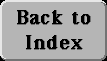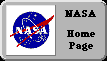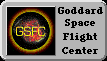Author and curator: David P. Stern, u5dps@lepvax.gsfc.nasa.gov
Last updated 4 February 1999